Copyright © Michael Richmond. This work is licensed under a Creative Commons License.

## Vectors and Vector Arithmetic

• Mathematical quantities come in two kinds:
1. scalars, which have magnitude only (but no direction)
2. vectors, which have magnitude and direction
• Unit vectors are just a bit of notation for describing the components of a vector
• The rules for adding, subtracting, multiplying and dividing scalars are the ones you learned in elementary school
• The rules for arithmetic on vectors are somewhat different
• The dot product of two vectors is a scalar. It is largest if the two vectors are parallel, and zero if the two vectors are perpendicular.
• The cross product of two vectors is another vector, which is always perpendicular to both the input vectors. It is largest if the two vectors are perpendicular, and zero if the two vectors are parallel.

Viewgraphs

•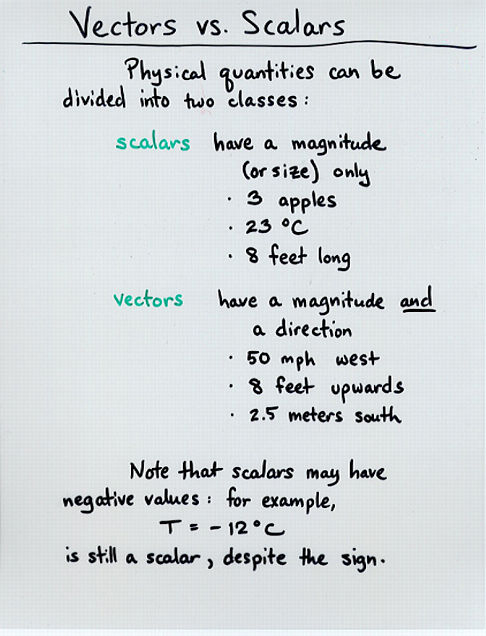Viewgraph 1
•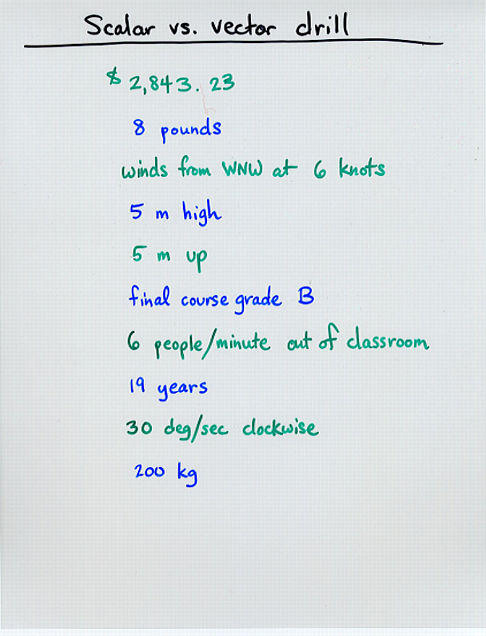Viewgraph 2
•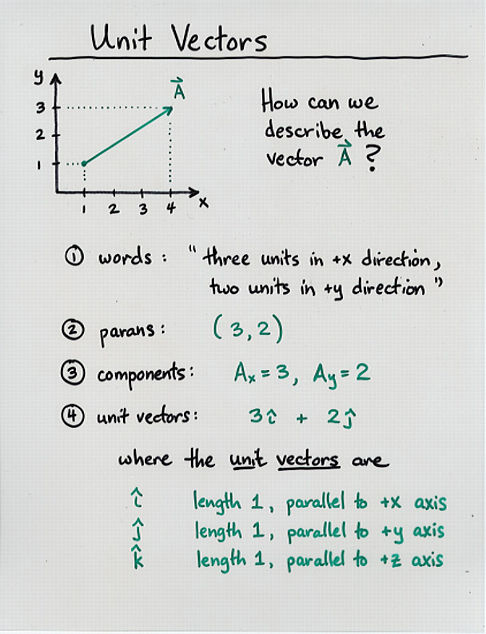Viewgraph 3
•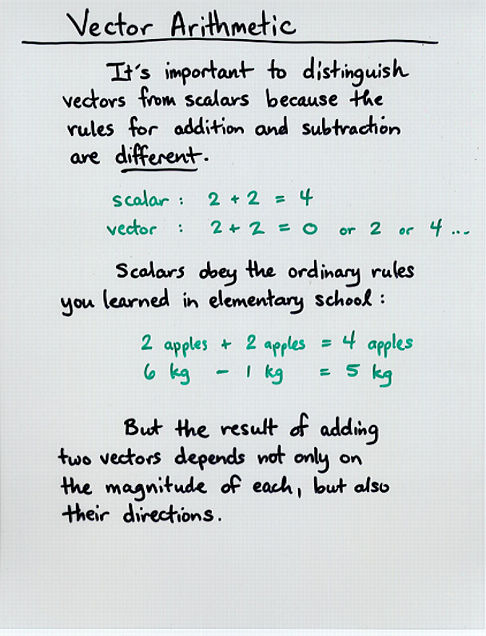Viewgraph 4
•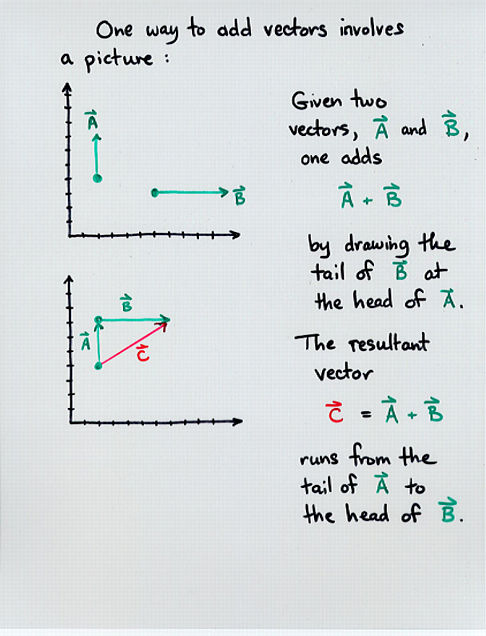Viewgraph 5
•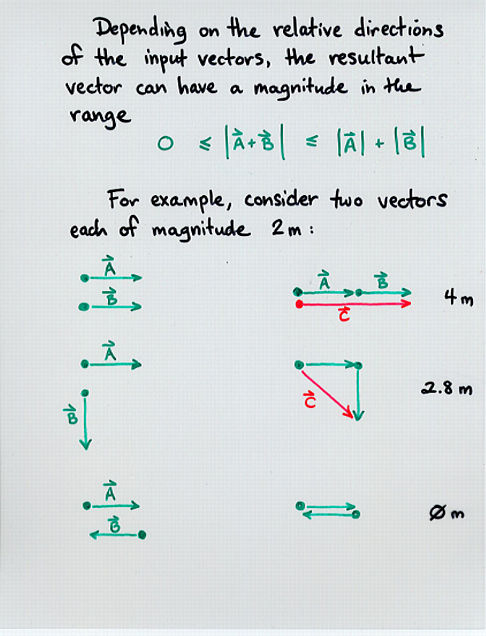Viewgraph 6
•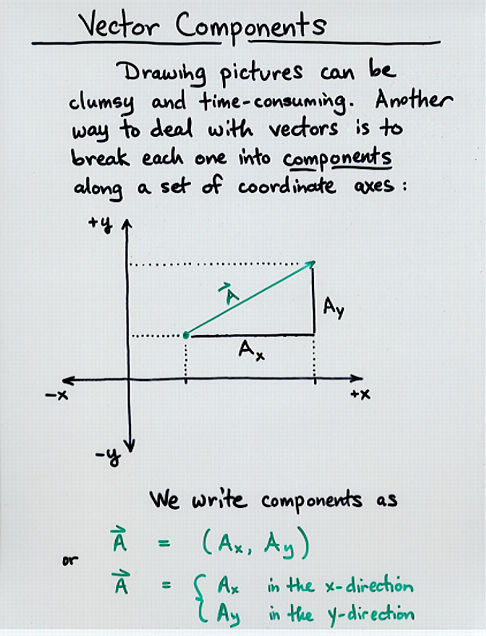Viewgraph 7
•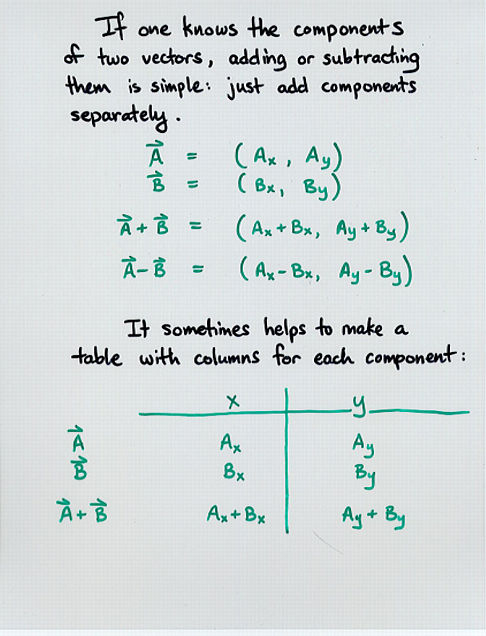Viewgraph 8
•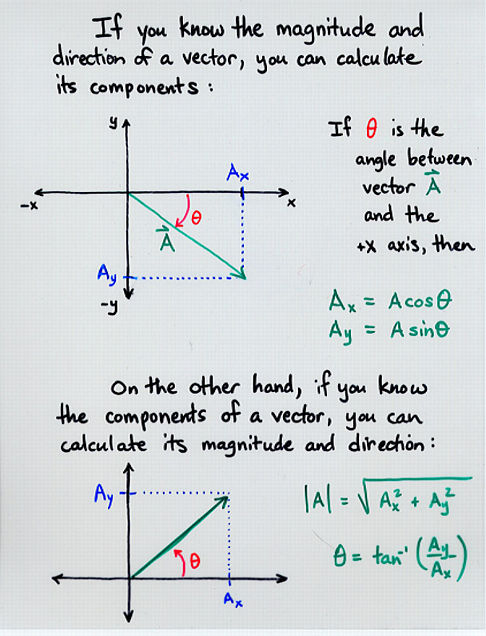Viewgraph 9
•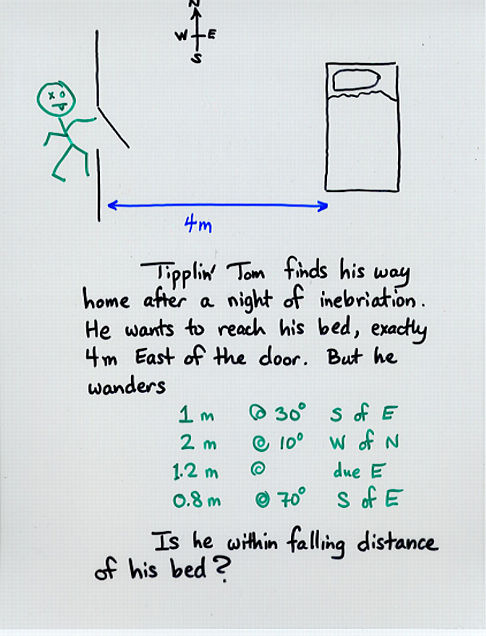Viewgraph 10
•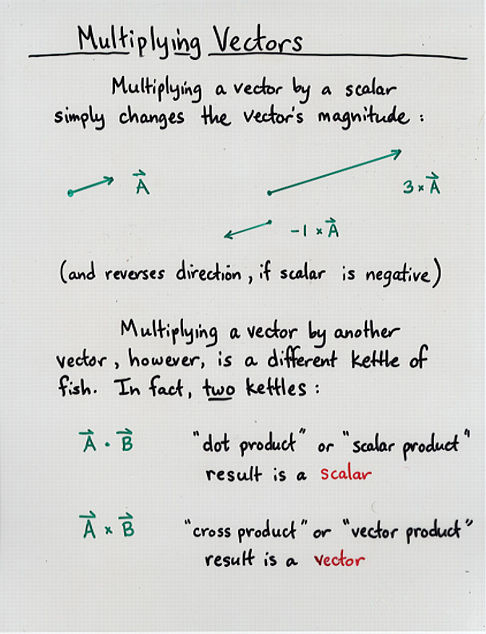Viewgraph 11
•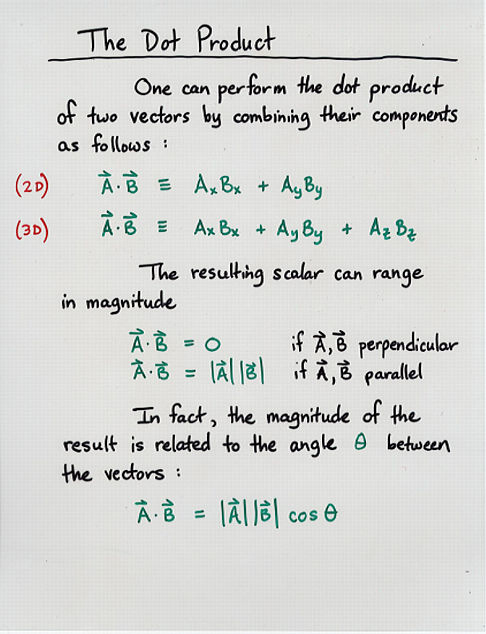Viewgraph 12
•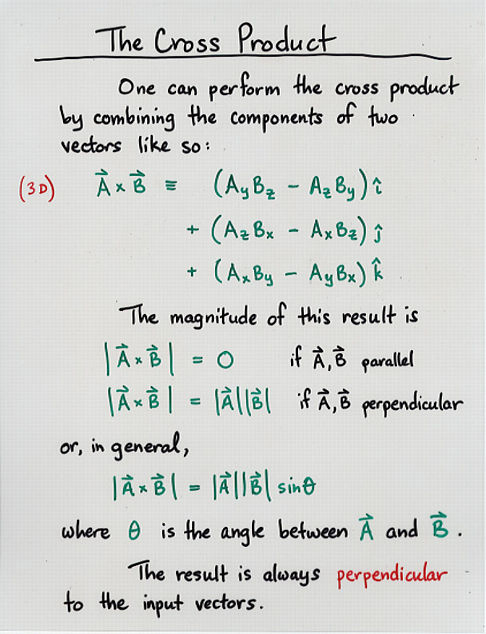Viewgraph 13
•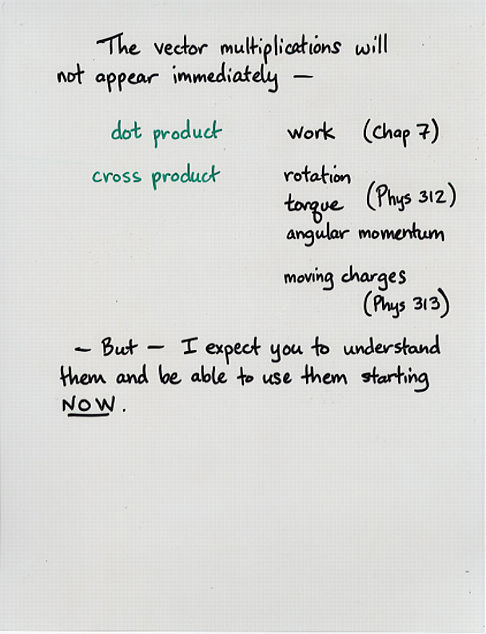Viewgraph 14Copyright © Michael Richmond. This work is licensed under a Creative Commons License.